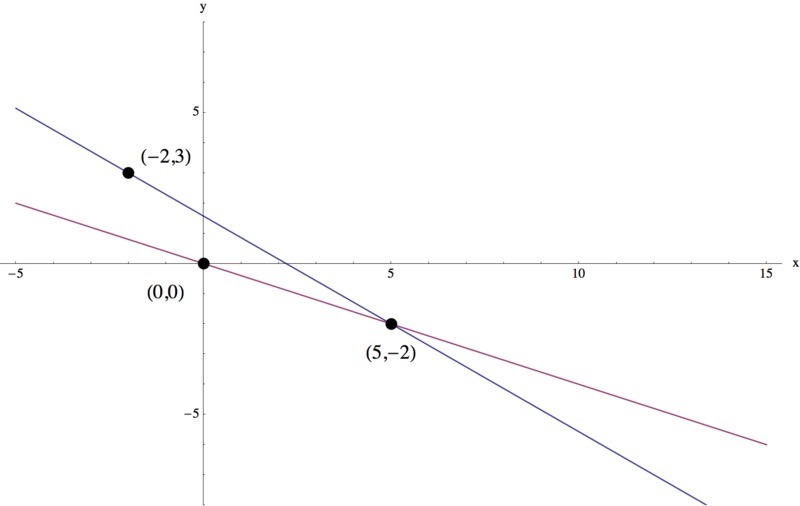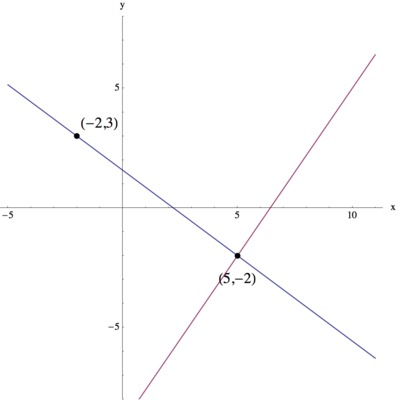# Find A System

Alignments to Content Standards: A-REI.C.6

Without graphing, construct a system of two linear equations where $(-2,3)$ is a solution to the first equation but not to the second equation, and where $(5,-2)$ is a solution to your system.

After you have created your system of equations, graph your system. Explain how your graph shows that your system satisfies the required conditions.

## IM Commentary

The purpose of this task is to encourage students to think critically about both the algebraic and graphical interpretation of systems of linear equations. They are expected to take what they know about solving systems of linear equations, and then reverse the usual process. This also serves as a good opportunity to ensure that students understand the difference between a single equation and a system where we consider two equations together, and in particular the phrase "solution to a system of equations."

There are two significantly different ways to find a possible second equation once we've found the first, and so those two methods are each outlined below (labeled (a) and (b)). Note that the line $x=c$ is not mentioned in the solution, though this is a perfectly viable equation to consider.

This task could be used as further practice and to deepen understanding after the material has already been introduced, or as an assessment task.

Also, this task asks the students to first produce a system of linear equations that meets given conditions without graphing, and then to consider their graphs. A simpler variation could be to get rid of this restriction. and allow students to first draw a graph as an aid in finding equations that fit the requirements. This would let students use a skill they have already learned and with which they may feel more comfortable.

For a similar more introductory variation, see 8.EE.8 The Intersection of Two Lines.

## Solution

As we are looking for two linear equations in $x$ and $y$, we know that we will be able to write our two equations in the form $y=mx+b$, where $m$ is the slope of the line and $b$ is the $y$-intercept.

Because we want $(5,-2)$ to be a solution to our system of equations, we know that this is a solution to both equations. As $(-2,3)$ is also a solution to the first equation, we now know that our first line contains the points $(5,-2)$ and $(-2,3)$. We can use these two points to find the slope of the line, by taking the difference in the $y$-coordinates and dividing by the difference in the $x$-coordinates:

$$m=\displaystyle\frac{3-(-2)}{(-2)-5}=-\frac{5}{7}$$

To find $b$, we use this value of $m$ and one of the points we want to be a solution, for example, $(-2,3)$. Substituting these known values into our generic form of $y=mx+b$, we have

$$3=-\frac{5}{7}(-2)+b$$ $$3=\frac{10}{7}+b$$ $$\frac{21}{7}-\frac{10}{7}=b$$ $$\frac{11}{7}=b.$$

So, our first equation is $\displaystyle y=-\frac{5}{7}x+\frac{11}{7}$.

There are many ways to find an equation to complete the system meeting the given conditions: (a) Pick any point that does not lie on the line $y=-5/7x+11/7$ and find the equation of the line through that point and $(5,-2)$, or (b) Pick any slope other than $-5/7$ and find the line with that slope that also goes through the point $(-5,2)$. Both methods are given below.

1. Consider the point $(0,0)$. Since $$0\neq -\frac{5}{7}(0)+\frac{11}{7}=\frac{11}{7},$$ $(0,0)$ is not a solution to our first equation. Hence, if we find the equation of the line through $(0,0)$ and $(5,-2)$, we will have found a second equation that fits the criteria for our system. We use these two points to find the slope of the line: $$m=\displaystyle\frac{(-2)-0}{5-0}=-\frac{2}{5}$$ To find $b$, we use this value of $m$ and one of the points we want to be a solution to the equation, for example, $(0,0)$. Substituting these known values into our generic form of $y=mx+b$, we have $$0=-\frac{2}{5}(0)+b$$ $$0=b$$ We also could have seen this because we chose the point $(0,0)$, and so the $y$-value at $x=0$ is 0.

So, our second equation is $\displaystyle y=-\frac{2}{5}x$, and our system of equations is $$\begin{cases} y=-\frac{5}{7}x+\frac{11}{7} \\ y=-\frac{2}{5}x \end{cases}$$ and we can verify that $(-2,3)$ is a solution to the first equation but not the second equation, and $(5,-2)$ is a solution to the system.

Next, we can graph these two equations on the same set of axes:This graph agrees with our system because we see that the two lines intersect at the point $(5,-2)$, and that the first equation also runs through the point $(-2,3)$ but that the second equation does not.

Note: the point $(0,0)$ was chosen here, but any other point that does not lie on the first line will do, so there could be infinitely many different answers!

2. We can pick any slope other that $-5/7$, and so let's pick a slope of $7/5$ and this will give us the line perpendicular to $\displaystyle y=-\frac{5}{7}x+\frac{11}{7}$.

Using this value of $m$ and the fact that we want $(5,-2)$ to be a solution, we can substitute these known values into our generic form of $y=mx+b$, and we have $$-2=\frac{7}{5}(5)+b$$ $$-2=7+b$$ $$-9=b$$

So, our second equation is $\displaystyle y=\frac{7}{5}x-9$, and our system of equations is $$\begin{cases} y=-\frac{5}{7}x+\frac{11}{7} \\ y=\frac{7}{5}x-9 \end{cases}$$ and we can verify that $(-2,3)$ is a solution to the first equation but not the second equation, and $(5,-2)$ is a solution to the system.

Next, we can graph these two equations on the same set of axes:This graph agrees with our system because we see that the two lines intersect at the point $(5,-2)$, and that the first equation also runs through the point $(-2,3)$ but that the second equation does not.